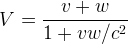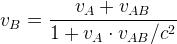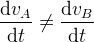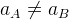## Errors in the Conclusions of Special Relativity

A false start does not necessarily mean a wrong ending. So let's have a look at the conclusions of Special Relativity.

The following equations are two of its conclusions:(a clock in motion runs slower)orThe first equation is about time dilation, which effectively puts a limit to the speed in the universe. If there is no limit for length, no limit for time, why do we need a limit for speed? If the numbering system in mathematics did not have infinity but used a large number as its limit, it would have been broken many times.

If the speed of light c is the speed limit in the universe, does not the c+v in the paper exceed that limit? If a conclusion breaks its precondition, does not that mean this theory is wrong?

The second equation is the velocity combination rule under Special Relativity. Under this transformation,orSo the principle of relativity is broken.

Only in a linear velocity combination rule, like the Galilean one, the influence of the reference frame can be completely removed by the differentiation of velocity with time, if the reference frame is in uniform motion.

If the principle of relativity works so well in this universe, which is introduced by Einstein himself, as can be seen from Part I, Section 05 of , why do we have to break it?

This breaking of the principle of relativity is not a surprise. The essence of Einstein's work is trying to build a bridge between relative motion and absolute motion. As relative motion is the base of Newtonian mechanics, which in turn is the foundation of the principle of relativity, any tiny change to the rule of relative motion will destroy the principle of relativity.

So, without going through its mathematical details, we can safely claim that Special Relativity is wrong.